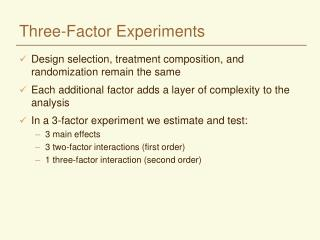DownloadDownload PresentationThree-Factor Experiments

# Three-Factor Experiments

Download Presentation## Three-Factor Experiments

- - - - - - - - - - - - - - - - - - - - - - - - - - - E N D - - - - - - - - - - - - - - - - - - - - - - - - - - -
##### Presentation Transcript

1. Three-Factor Experiments • Design selection, treatment composition, and randomization remain the same • Each additional factor adds a layer of complexity to the analysis • In a 3-factor experiment we estimate and test: • 3 main effects • 3 two-factor interactions (first order) • 1 three-factor interaction (second order)

2. Same Song - Second Verse... • Construct tables of means • Complete an ANOVA table • Perform significance tests • Compute appropriate means and standard errors • Interpret the results

3. In General... • We have ‘a’ levels of A, ‘b’ levels of B, and ‘c’ levels of C • Total number of treatments will be a x b x c • Total number of plots will be a x b x c x r • SSTot = • Where • i= 1 to a • j = 1 to b • k = 1 to c • l = 1 to r

4. Table of Means for Treatments Treatment Means over Reps A1B1C1 T111 A1B1C2 T112 A1B1C... T11.. A1B1Cc T11c A1B2C1 T121 A1B2C2 T122 A1B2C... T12.. A1B2Cc T12c .... AaBbCc Tabc Compute a mean over replications for each treatment. Total number of treatments = a x b x c

5. Table of Block Means • If there are blocks… Block I II III Mean R1 R2 R3

6. Table for Main Effects Level 1 2 ... f Factor A A1 A2 ... Aa Factor B B1 B2 ... Bb Factor C C1 C2 ... Cc Where A1 represents the mean of all of the treatments involving Factor A at level 1, averaged across all of the other factors.

7. First-Order Interactions Factor B Factor A 1 2 ... b 1 T11. T12. ... T1b. 2 T21. T22. ... T2b. ... ... ... ... ... a Ta1. Ta2. ... Tab. Compute a table such as this for each first order interaction: A x B A x C B x C

8. ANOVA Table (fixed model) Source df SS MS F Total rabc-1 SSTot= Block r-1 SSR= MSR= FR SSR/(r-1) MSR/MSE Main Effects A a-1 SSA= MSA= FA SSA/(a-1) MSA/MSE B b-1 SSB= MSB= FB SSB/(b-1) MSB/MSE C c-1 SSC= MSC= FC SSC/(c-1) MSC/MSE

9. ANOVA Table Continued... Source df SS MS F First Order Interactions AB (a-1)(b-1) SSAB= MSAB FAB AC (a-1)(c-1) SSAC= MSAC FAC BC (b-1)(c-1) SSBC= MSBC FBC 3-Factor Interaction ABC (a-1)(b-1)(c-1) SSABC= MSABC FABC Error (r-1)(abc-1) SSE= MSE SSTot-SSR-SSA-SSB-SSC -SSAB-SSAC-SSBC-SSABC

10. Standard Errors Factor Std Err of Mean Std Err of Difference A MSE/rbc 2MSE/rbc B MSE/rac 2MSE/rac C MSE/rab 2MSE/rab AB MSE/rc 2MSE/rc AC MSE/rb 2MSE/rb BC MSE/ra 2MSE/ra ABC MSE/r 2MSE/r

11. Interpretation • Depends on the outcome of the F tests for main effects and interactions • If the 3-factor (AxBxC) interaction is significant • None of the factors are acting independently • Summarize with 3-way table of means for each treatment combination • If 1st order interactions are significant (and not the 3-factor interaction) • Neither of the main effects are independent • Summarize with 2-way table of means for significant interactions • If Main Effects are significant (and not any of the interactions) • Summarize significant main effects with a 1-way table of factor means

12. Example • Seed yield of chickpeas • Study the effect of three production factors: • Variety (2) • Phosphorus Fertilization (3) • None, 25 kg/ha, 50 kg/ha • Weed Control (2) • None, Herbicide • Using an RBD design in three blocks

13. ANOVA Source df SS MS F Total 35 1936.75 Block 2 270.17 135.08 5.93** Variety (V) 1 306.25 306.25 13.44** Phosphorus (P) 2 32.00 16.00 .70 Herbicide (W) 1 12.25 12.25 .54 V x P 2 18.67 9.33 .41 V x W 1 283.36 283.36 12.44** P x W 2 468.67 234.33 10.29** V x P x W 2 44.22 22.11 .97 Error 22 501.16 22.78

14. Herbicide Variety None Some V1 56.89 52.44 V2 57.11 63.89 *Standard error = 1.59 Phosphorus Herbicide None 25 kg/ha 50 kg/ha None 60.00 57.83 53.17 Some 52.50 58.67 63.33 *Standard error = 1.95 Mean seed yield (kg/plot) of chick-peas at three levels of phosphorus fertilization with and without herbicide Means and Standard Errors Mean seed yield (kg/plot) from two varieties of chick-peas with and without herbicide

15. Interpretation • The effect of herbicide depended on variety • The addition of herbicide reduced the yield for variety 1 • The yield of variety 2 was increased by the use of herbicide • Response to added phosphorus depended on whether or not herbicide was used • If no herbicide, seed yield was reduced when phosphorus was added • However, seed yield increased when phosphorus was added in addition to herbicide

16. A Picture is Worth a Thousand Words Herbicide x Variety Interactions Phosphorus x Herbicide Interactions V2 64 64 62 62 60 60 Yield Yield 58 58 56 56 54 54 V1 52 52 0 25 50 Some None Herbicide Phosphorus in kg/ha Without Herbicide With Herbicide